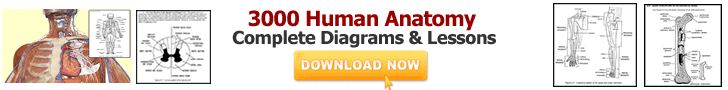## Cool second grade math worksheetsKids can use our 2nd grade math worksheets to practice their math skills. This site does not contain math lessons only, but all of the 2nd grade math lessons are followed by worksheets to practice the skills learned in the lessons. Sample worksheets are given below to print:

 Skipped Hundreds ChartNumbers in WordsSkip Counting WorksheetPlace Value WorksheetsRounding Numbers2nd Grade AdditionSubtraction WorksheetsGeometric ShapesPattern WorksheetsFraction WorksheetsMoney WorksheetsTelling Time Worksheets@import url(http://www.google.com/cse/api/branding.css);Custom Search